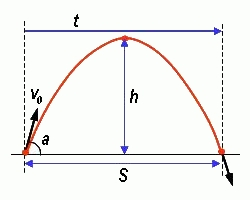# Projection

Calculation of unknown parameters of projection using known parameters. Parameters are duration, maximum height, distance, initial velocity and angle.

### This page exists due to the efforts of the following people:

#### Timur

• Article : Projection - Author, Translator ru - en
• Calculator : Projection - Author, Translator ru - en
Created: 2011-07-23 13:56:52, Last updated: 2021-02-12 11:24:22This calculator helps to determine parameters of projection, or ballistic motion. The parameters of projection, as shown on picture, are:
distance ,
maximum height ,
flight duration ,
initial angle ,
initial velocity .

The main equations are:

These formulae are produced from accelerated motion equations assuming there is no acceleration along with the x-axis and only gravity acceleration "g" along the y-axis.

The calculator can find unknown parameters for any pair of known parameters.
It calculates the flight duration, maximum height, and travel distance of the projection from the initial velocity and angle. Or it calculates the initial velocity, travel distance, and flight duration of the projection from the initial angle and maximum height. Or, etc. The only illegal combination of parameters is the maximum height and flight duration. Given only these, it is impossible to find others.

Gravitational acceleration, m/s2
Digits after the decimal point: 2
Initial velocity (m/s)

Angle (degrees)

Duration (secs)

Distance (m)

Maximum height (m)

URL copied to clipboard
PLANETCALC, Projection